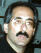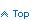# Populate an Excel Worksheet from .NET using the Excel object

You can format your data as a TAB delimited string, use the Excel object to start an instance of Microsoft Excel, select the worksheet and range to populate, and paste in your data.

To use the Excel object you must first set a COM reference to the Microsoft Excel Object Library which is an ActiveX component. Behind the scenes .NET uses the Interop.Excel DLL to talk to Excel. This DLL must be distributed with your application.

An issue exists with the Excel object when the user closes your application before closing the instance of Excel that you started. This instance becomes an orphaned process - i.e. when you look at the Processes tab in Windows Task Manager you will see a process exists for Excel.Exe yet there will be no visible Excel applications or windows present.

The VB.NET code below shows how to populate Excel and account for this scenario.

Module level variables in your form's Declarations section:

```    '
' Variables to refer to the Excel Object and process.
'
Private myExcel As Excel.Application
Private myExcelPID As Integer
Private myDataSet As DataSet
```

Start Excel and get its process ID:

```    '
' Verify the Excel file to populate exists.
'
Dim strExcelFile As String = "C:\MyApp\MyExcel.xls"
If Not IO.File.Exists(strExcelFile) Then Return
'
' Start Microsoft Excel and make it visible.
'
myExcel = New Excel.Application

If myExcel Is Nothing Then
Throw (New Exception("Unable to Start Microsoft Excel."))
Else
myExcel.Visible = True
myExcel.WindowState = Excel.XlWindowState.xlMaximized
'
' Get the process ID of the Excel instance. This is used
' in the Closing Event to prevent orphaned Excel processes.
'
Dim aProcesses() As Process = Process.GetProcesses
Dim i As Integer

For i = 0 To aProcesses.GetUpperBound(0)
If aProcesses(i).MainWindowHandle.ToString = myExcel.Hwnd.ToString Then
myExcelPID = aProcesses(i).Id
Exit For
End If
Next
End If
```

Open the XLS file and activate the worksheet to populate:

```    Dim strWorkSheetName As String = "MyData"
Dim sbExcelData As System.Text.StringBuilder
Dim aWorkbook As Excel.Workbook
Dim aWorkSheet As Excel.Worksheet

aWorkbook = myExcel.Workbooks.Open(strExcelFile)
aWorkSheet = CType(aWorkbook.Sheets(strWorkSheetName), Excel.Worksheet)
aWorkSheet.Activate()
```

Define the Excel Range to populate:

```    '
' Assume the data to send to Excel is in a dataset and it has 5 columns
' and will fill Excel starting with column C and row 4.
'
Dim intRows As Integer = myDataSet.Tables("MyTable").Rows.Count - 1
Dim strRangeString As String = "C4:G" & intRows.ToString
Dim aRange As Excel.Range = aWorkSheet.Range(strRangeString)
'
' Remove any existing data from Excel.
'
aRange.ClearContents()
```

Convert the data in the dataset to a string to paste into Excel:

```    If Not ConvertDataForExcel(sbExcelData) Then
Return
End If

Clipboard.SetDataObject(sbExcelData.ToString, False)
aRange.Select()
aWorkSheet.Paste()
'
' Unselect the range.
'
aRange = aWorkSheet.Range("A1:A1")
aRange.Select()
```

Convert the data to a TAB delimited string. The System.Text.StringBuilder is used for performance:

```    Private Function ConvertDataForExcel( _
ByRef theExcelData As System.Text.StringBuilder) As Boolean
Try
Dim i As Integer
Dim adr As DataRow
Dim adt As DataTable = myDataSet.Tables("MyTable")

For Each adr In adt.Rows
For i = 0 to 4
'
' Convert the data and fill the string. Null values become blanks.
'
If adr(i) Is DBNull.Value Then
theExcelData.Append("")
Else
theExcelData.Append(adr(i).ToString)
End If
theExcelData.Append(vbTab)
Next
'
' Add a line feed to the end of each row.
'
theExcelData.Append(vbCrLf)
Next

Catch ex As Exception
' Display an error message.
Return False
End Try

End Function
```

Code to prevent orphaned Excel processes. First quit Excel then tell the InterOp layer to release the COM component:

```    Private Sub Form1_Closing(ByVal sender As Object, _
ByVal e As System.ComponentModel.CancelEventArgs) Handles MyBase.Closing

Try
'
' Close Excel.
'
myExcel.Quit()
System.Runtime.InteropServices.Marshal.ReleaseComObject(myExcel);

Finally
myExcel = Nothing
'
' If this screen is closed before the Excel application
' there will be an orphan Excel process.
'
Try
'
' Look for an Excel process matching the one we started.
'
Dim aProcesses() As Process = Process.GetProcesses
Dim aProcess As Process
Dim i As Integer

For i = 0 To aProcesses.GetUpperBound(0)
If aProcesses(i).Id = myExcelPID Then
aProcess = aProcesses(i)
Exit For
End If
Next

Try
'
' If we found one see if it has exited.
'
If Not (aProcess Is Nothing) Then
If aProcess.ProcessName.ToUpper = "EXCEL" Then
If Not aProcess.HasExited Then
'
' It hasn't exited. If it has no main window we
' have an orphaned process that we should kill.
'
If aProcess.MainWindowHandle.ToString = "0" Then
aProcess.Kill()
Else
'
' The Excel process has a main window meaning
' the Excel file is still open. Tell the user to
' close Excel before closing this screen.
'
e.Cancel = True
MessageBox.Show( _
"Please close Excel before closing this screen.", _
"Warning", _
MessageBoxButtons.OK, MessageBoxIcon.Exclamation)
End If
End If
End If
End If

Catch

End Try

Catch ex As Exception

End Try
End Try
End Sub
```

Here is a similar sample written in C#. This code is encapsulated in a separate class. Examples on how to call use this class are shown at the bottom of this page. This code dynamically determines the Excel range to use by decoding the Tab delimited data string passed to it. It uses one worksheet to store data and a second worksheet to store the database query that produced the data that was stored.

```namespace iExcelClass
{
public class iExcel
{
private Excel.Application _mExcelApp;
private Excel.Workbook _mWorkbook;
private Excel.Worksheet _mWorkSheet;
private int _mintSheet1Row = 1;
private int _mintSheet2Row = 1;
private int _mExcelPID = 0;
private bool _mblnExcelVisible = false;
private string _mstrXLSFileFullName = "";
private string _mstrWorkSheetName = "MyWkShtName";

public iExcel()
{
}

public void StartExcel(string XLSFileFullName)
{
//
// Start Microsoft Excel, make it visible, add a worksheet.
//
_mExcelApp = new Excel.Application();

if (_mExcelApp == null)
{
throw (new Exception("Unable to Start Microsoft Excel."));
}

_mstrXLSFileFullName = XLSFileFullName;
_mExcelApp.Visible = _mblnExcelVisible;
_mExcelApp.WindowState = Excel.XlWindowState.xlMinimized;
_mWorkbook = _mExcelApp.Workbooks.Add(Excel.XlWBATemplate.xlWBATWorksheet);
_mWorkSheet = (Excel.Worksheet) _mWorkbook.Worksheets.Add(
Type.Missing,
Type.Missing,
Type.Missing,
Type.Missing);
_mWorkSheet.Name = _mstrWorkSheetName;
_mExcelApp.SheetsInNewWorkbook = 1;
_mWorkSheet.Activate();
//
// Get the Excel instance's process ID...used later
// to prevent orphaned Excel processes.
//
Process [] aProcesses = Process.GetProcesses();

for (int i = 0; i <= aProcesses.GetUpperBound(0); i++)
{
if (aProcesses[i].MainWindowHandle.ToString() == _mExcelApp.Hwnd.ToString())
{
_mExcelPID = aProcesses[i].Id;
break;
}
}
}

public void AddRow(string TabDelimitedData)
{
//
// Decipher the input string's characteristics to calculate the
// Excel Range to paste it into.
//
string [] cols = TabDelimitedData.Split('\t');
if (cols.Length == 0)
{
return;
}

int i = 1;
string strCol = "";

while(i * 26 <= cols.Length)
{
i++;
}

if (i == 1)
{
strCol = Convert.ToChar(64 + cols.Length).ToString();
}
else
{
strCol = Convert.ToChar(64 + i - 1).ToString();
strCol += Convert.ToChar(64 + cols.Length - ((i-1)*26)).ToString();
}
string strRangeString =
"A" + _mintSheet1Row.ToString() + ":" + strCol + _mintSheet1Row.ToString();

//
// Define the Range, clear it, paste the data.
//
Excel.Range _mRange = _mWorkSheet.get_Range(strRangeString, Type.Missing);
_mRange.ClearContents();
_mRange.Select();
Clipboard.SetDataObject(TabDelimitedData, false);
_mWorkSheet.Paste(Type.Missing, Type.Missing);

//
// Unselect the range.
//
_mRange = _mWorkSheet.get_Range("A1:A1", Type.Missing);
_mRange.Select();
_mintSheet1Row++;
}

public void AddQueryDetails(string TabDelimitedData)
{
Excel.Worksheet _WorkSheet = ((Excel.Worksheet) _mWorkbook.Sheets);
_WorkSheet.Name = "Query Details";
_WorkSheet.Activate();

//
// Decipher the input string's characteristics to calculate the
// Excel Range to paste it into.
//
string [] cols = TabDelimitedData.Split('\t');
if (cols.Length == 0)
{
return;
}

string strCol = Convert.ToChar(64 + cols.Length).ToString();
string strRangeString =
"A" + _mintSheet2Row.ToString() + ":" + strCol + _mintSheet2Row.ToString();

//
// Define the Range, clear it, paste the data.
//
Excel.Range _Range = _WorkSheet.get_Range(strRangeString, Type.Missing);
_Range.ClearContents();
_Range.Select();
Clipboard.SetDataObject(TabDelimitedData, false);
_WorkSheet.Paste(Type.Missing, Type.Missing);

//
// Unselect the range.
//
_Range = _WorkSheet.get_Range("A1:A1", Type.Missing);
_Range.Select();
_mintSheet2Row++;
}

public void SaveFile()
{
//
// Overwrite existing XLS file without alerting the user.
//
_mExcelApp.DisplayAlerts = false;

_mExcelApp.ActiveWorkbook.SaveAs(
_mstrXLSFileFullName,
Excel.XlFileFormat.xlXMLSpreadsheet,
Type.Missing,
Type.Missing,
Type.Missing,
Type.Missing,
Excel.XlSaveAsAccessMode.xlNoChange,
Type.Missing,
Type.Missing,
Type.Missing,
Type.Missing,
Type.Missing);
}

public void CloseExcel()
{
try
{
//
// Close the Excel application.
// Tell the stupid interop layer to release the object.
//
_mExcelApp.Quit();
System.Runtime.InteropServices.Marshal.ReleaseComObject(_mExcelApp);
}

finally
{
//
// Prevent an orphaned Excel process by forcibly killing it.
//
_mExcelApp = null;
Process [] aProcesses  = Process.GetProcesses();
Process aProcess = null;

try
{
//
// Look for an Excel process matching the one we started.
//
for (int i = 0; i <= aProcesses.GetUpperBound(0); i++)
{
if (aProcesses[i].Id == _mExcelPID)
{
aProcess = aProcesses[i];
break;
}
}

try
{
//
// If we found a matching Excel proceess with no main window
// associated main window, kill it.
//
if (aProcess != null)
{
if (aProcess.ProcessName.ToUpper() == "EXCEL")
{
if (!aProcess.HasExited)
{
if (aProcess.MainWindowHandle.ToString() == "0")
{
aProcess.Kill();
}
else
{
//
// The Excel process has a main window meaning the Excel
// file is still open. Tell the user to close Excel.
//
MessageBox.Show("Please close Excel before closing this screen.",
"Warning", MessageBoxButtons.OK, MessageBoxIcon.Exclamation);
}
}
}
}
}
catch {}
}
catch {}
}
}

//
// Public Properties
//
public string XLSFileFullName
{
get { return _mstrXLSFileFullName; }
}

public string WorkSheetName
{
get { return _mstrWorkSheetName; }
set { _mstrWorkSheetName = value; }
}

public bool ExcelVisible
{
get { return _mblnExcelVisible; }
set { _mblnExcelVisible = value; }
}
}
}
```
>

Sample Calls:

```   iExcelClass.iExcel oExcel = new iExcelClass.iExcel();

oExcel.WorkSheetName = "MyWorkSheet";
oExcel.ExcelVisible = false;
oExcel.StartExcel(@"C:\...\MyXLSFile.xls");
//
// Add a rows of data to Excel. Datastring is a Tab delimited string
// such as: "aaa\tbbb\tccc"
//
for ...
{
oExcel.AddRow(DataString);
}

//
// Add details of the query that produced the data to a second worksheet.
//
oExcel.AddQueryDetails("Date Run\tDatabase Name\tQuery Run");
oExcel.AddQueryDetails("06-01-2004\tProduction\tSelect * FROM Table1");

oExcel.SaveFile();
oExcel.CloseExcel();
```About TheScarms

Sample code
version info

 If you use this code, please mention "www.TheScarms.com"

© Copyright 2019 TheScarms